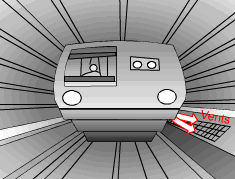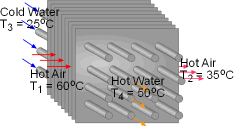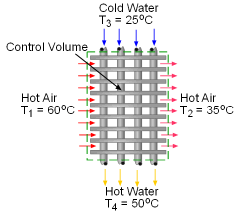Ch 7. Exergy Analysis Multimedia Engineering Thermodynamics Exergy ExergyChange ExergyBalance(1) ExergyBalance(2)
 Chapter 1. Basics 2. Pure Substances 3. First Law 4. Energy Analysis 5. Second Law 6. Entropy 7. Exergy Analysis 8. Gas Power Cyc 9. Brayton Cycle 10. Rankine Cycle Appendix Basic Math Units Thermo Tables Search eBooks Dynamics Fluids Math Mechanics Statics Thermodynamics Author(s): Meirong Huang Kurt Gramoll ©Kurt GramollTHERMODYNAMICS - CASE STUDY IntroductionProblem GraphicSchematic of the Heat Recovery System When a train in a subway approaches the station, it releases heat to its surrounding air when it brakes to stop. The heated air is exhausted through the vents under the station island. An ideal to reuse this waste heat is to design a waste heat recovery system. In this system, cold water is heated by the exhausted air. To evaluate the recovery system, exergy analysis of this system should be done. What is known: 200 kg/s air at 60oC enters the heat recovery system. Air leaves the heat recovery system at 35oC. Cold water at 25oC is sent to the heat recovery system and leaves at 50oC. Ambient temperature is 25oC.Take the Recover System as a Control Volume Question Determine the mass flow rate of the cold water. How much exergy is destroyed during the heat exchange process in the heat recovery system? Determine the second-law efficiency of the heat recovery system. Approach Take heat recovery system as a control volume. The heat recovery system is a heat exchanger with two unmixed fluid stream. Use energy balance of the control volume to determine the cold water mass flow rate. Use the exergy balance to determine the exergy destruction. The second-law efficiency can be determined from the definition of the second-law efficiency of heat exchanger with two unmixed fluid stream.